Refer to our Texas Go Math Grade 2 Answer Key Pdf to score good marks in the exams. Test yourself by practicing the problems from Texas Go Math Grade 2 Module 6 Assessment Answer Key.

Vocabulary

Use a word from the box to complete the sentence.

regroup

Question 1.
When adding 37 + 16. ____________________ 10 ones to make 1 ten. (p.198)
When adding 37 + 16. Regroup 10 ones to make 1 ten. (p.198)
37 + 16
30 + 7
16 is written as 3 + 13
7 + 3 = 10
30 + 10 + 13 = 53
37 + 16 = 53

Concepts and Skills

Break apart the addends to find the sum. (TEKS 2.4.B)

Question 2.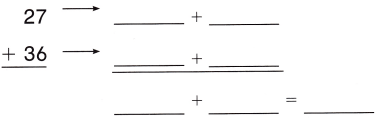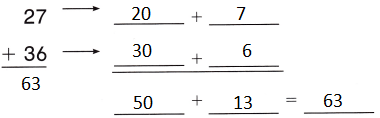Explanation:
27 + 36
20 + 7 =
20 + 3 + 4
23 + 4 + 36 = 40 + 23 = 63
27 + 36 = 63

Question 3.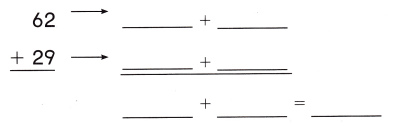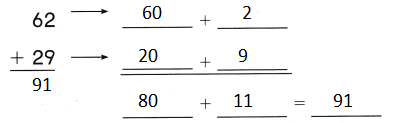Explanation:
80 + 11 = 91
62 + 29 = 91
The tens and ones are added separately

Draw quick pictures to help you solve. Write the sum. (TEKS 2.4.B)

Question 4.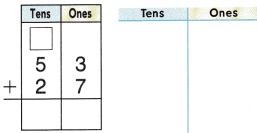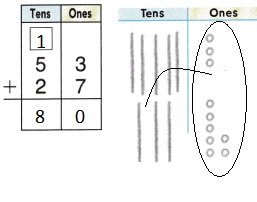Explanation:
ones are converted to tens
10 ones = 1 tens

Question 5.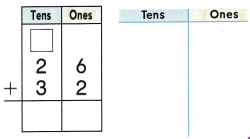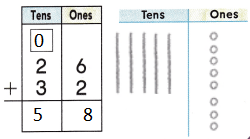Explanation:
ones are converted to tens
10 ones = 1 tens

Fill in the bubble for the correct answer choice.

Question 6.
The baker sold 9 muffins on Friday and 38 muffins on Saturday. How many muffins were sold on those two days? (TEKS 2.4.B)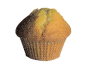(A) 49
(B) 41
(C) 47
Explanation:
The baker sold 9 muffins on Friday and 38 muffins on Saturday.
38 + 9
9 is written as 2 + 7
40 + 7 = 47
47 muffins were sold on those two days

Question 7.
Mr. O’Connor asked Joey to solve 58 + 24 by making one addend the next tens number. Which addition problem can Joey use to find the sum? (TEKS 2.4.B)(A) 60 + 30
(B) 60+ 22
(C) 60 + 24
Explanation:
Mr. O’Connor asked Joey to solve 58 + 24 by making one addend the next tens number.
60 + 22 = 82
60+ 22 addition problem can Joey use to found the sum

Question 8.
Edna has 15 color pencils. She has I more color pencil than Manny. How many color pencils do Edna and Manny have? (TEKS 2.4.B)
(A) 29
(B) 16
(C) 39
Explanation:
Edna has 15 color pencils.
She has I more color pencil than Manny.
15 – 1 = 14
15 + 14 = 29
29 color pencils that Edna and Manny have

Question 9.
Amy picked 29 strawberries. Her mother picked 46 strawberries. How many strawberries did they pick? (TEKS 2.4.B )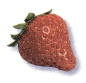(A) 75
(B) 65
(C) 66